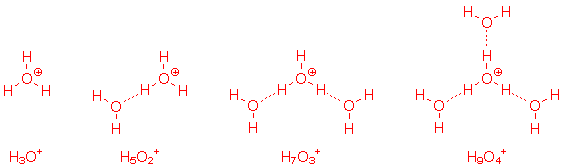MadSci Network: Chemistry Query:

Re: Why is the pH of 12 Molar HCl 0 and not -1.07?

Date: Thu May 7 16:41:42 1998
Posted By: Dan Berger, Faculty Chemistry/Science, Bluffton College
Area of science: Chemistry
ID: 893835592.Ch
Message:

Why is the pH of 12 Molar HCl 0 and not -1.07?

I understand that no chemical can have a pH less than 0 in an aqeous solution, but if that is the case, then how can a 12M aqueous solutoin of HCl exist if the mathematically determined pH is -1.08?

pH is not determined mathematically; it is determined by a pH meter. Chemistry is an experimental science, in spite of the best efforts of the theoreticians!

pH measures the ability of a solution to transfer protons, or, more formally, the activity of H+. pH is the negative logarithm of the activity of H+, which is not formally the same as the concentration.

At relatively low concentrations of acid, the activity of H+ is about equal to the concentration. But by the time you reach 12 moles per liter, the concentration is no longer low and the approximation that aH+ = [H+] no longer holds.

The reason aqueous solutions can't have a pH lower than zero is that, at that point, the limiting factor is no longer the availability of protons but the rate at which they can be transferred from "hydronium ion," the active acid in any aqueous solution.

Hydronium ion is usually represented by the formula H3O+, but its true formula is not certain. I've seen claims that the average aggregate is actually H5O2+ or even H7O3+ or H9O4+; see the picture below. Hydrogen bonds are represented by dashed lines.Current Queue | Current Queue for Chemistry | Chemistry archives